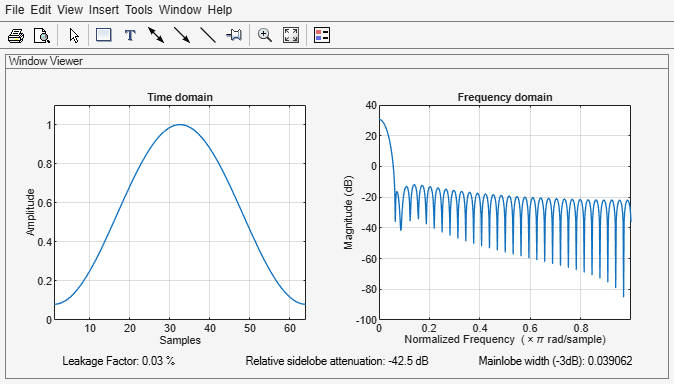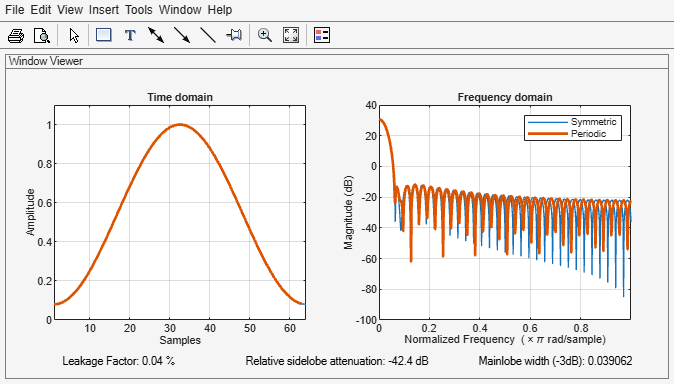Documentation

### This is machine translation

Mouseover text to see original. Click the button below to return to the English version of the page.

Note: This page has been translated by MathWorks. Click here to see
To view all translated materials including this page, select Country from the country navigator on the bottom of this page.

# hamming

Hamming window

## Syntax

``w = hamming(L)``
``w = hamming(L,sflag)``

## Description

example

````w = hamming(L)` returns an `L`-point symmetric Hamming window.```

example

````w = hamming(L,sflag)` returns a Hamming window using the window sampling specified by `sflag`.```

## Examples

collapse all

Create a 64-point Hamming window. Display the result using `wvtool`.

```L = 64; wvtool(hamming(L))```Design two Hamming windows:

• The first window has N = 64 and is symmetric.

• The second window has N = 63 and is periodic.

Display the two windows.

```Hs = hamming(64,'symmetric'); Hp = hamming(63,'periodic'); wvt = wvtool(Hs,Hp); legend(wvt.CurrentAxes,'Symmetric','Periodic')```## Input Arguments

collapse all

Window length, specified as a positive integer.

Data Types: `single` | `double`

Window sampling method, specified as:

• `'symmetric'` — Use this option when using windows for filter design.

• `'periodic'` — This option is useful for spectral analysis because it enables a windowed signal to have the perfect periodic extension implicit in the discrete Fourier transform. When `'periodic'` is specified, the function computes a window of length `L` + 1 and returns the first `L` points.

## Output Arguments

collapse all

Hamming window, returned as a column vector.

## Algorithms

The following equation generates the coefficients of a Hamming window:

`$w\left(n\right)=\begin{array}{cc}0.54-0.46\mathrm{cos}\left(2\pi \frac{n}{N}\right),& 0\le n\le N\end{array}.$`

The window length L = N + 1.

 Oppenheim, Alan V., Ronald W. Schafer, and John R. Buck. Discrete-Time Signal Processing. Upper Saddle River, NJ: Prentice Hall, 1999.